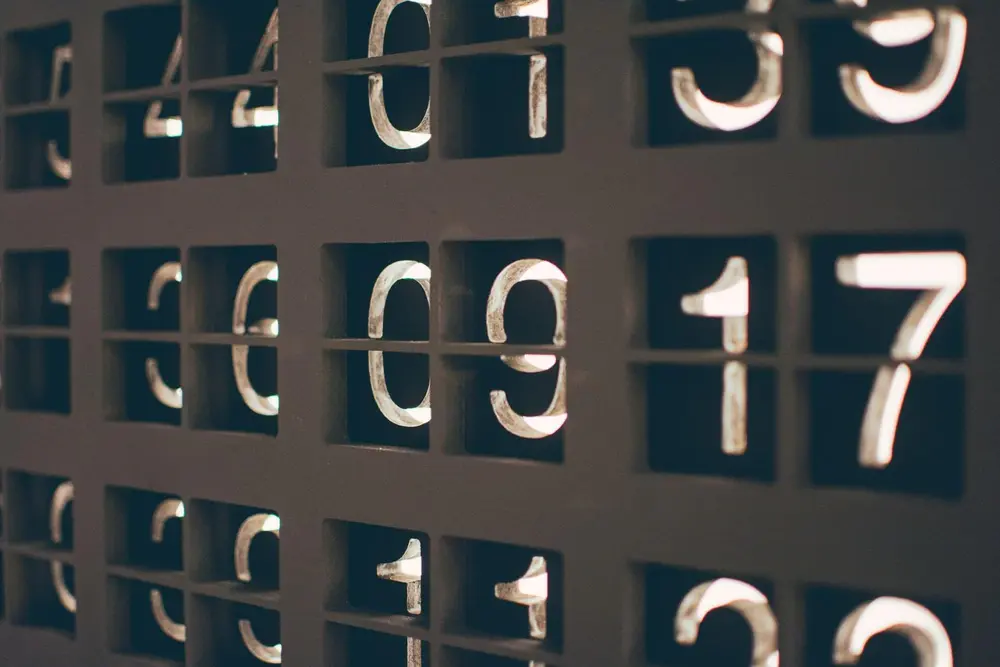# Fractions

Fractions show a number in relation to how many parts there are. They are mostly used to show a number smaller than 1. However, whole numbers can be expressed as fractions as well. You can easily use fractions on most new calculators. However, you should still understand how they work.

A Fraction is expressed as: numerator / denominator

You have to have a common denominator (this is most easily done by multiplying the two denominators together but remember to do the same to the numerator). 1/3 + 2/5 = 5/15 + 6/15 = 11/15
Subtraction
Same method as with addition. 1/2 - 1/3 = 3/6 - 2/6 = 1/6
Multiplication
Here you just multiply the numerators together and the denominators together. 1/4 × 1 1/2 = 1/4 × 3/2 = 3/8
Division
To do this you have to turn the divisor upside down. Then you multiply. 2 1/4 ÷ 1/4 = 9/4 ÷ 1/4 = 9/4 × 4/1 = 36/4 = 9

Number

Number

Number

Number

Number

Number